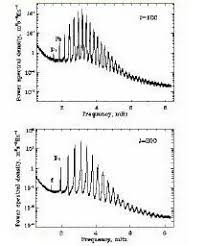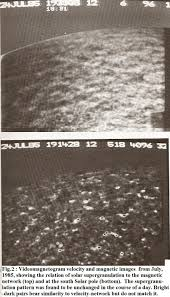## Wednesday, September 18, 2019

### Solar Oscillations RevisitedA computer-generated image of some of the 10 million modes of acoustic waves on the Sun (red indicating receding wave fronts, and blue approaching).

The phenomenon  and measurement -interpretation of solar oscillations being a relatively new field, mainly developed in the last forty-five years, few lay folk are aware of it.. However, plumbing the Sun's depths to investigate its different modes of vibration allows hitherto unknown tools to be applied to many types of solar  predictions.

The first reckoning of non-radial oscillations arrived ca. 1968 with the work of Frazier who made two-dimensional plots of wavenumber vs. frequency or (k -w) diagrams. Several peaks of amplitude were found and it was suggested that these corresponded to the fundamental and first overtones for the solar envelope. Interestingly the patterns of solar oscillations - namely the acoustic or "p-modes" resemble those detected on drum heads by computer holography.

The Sun is clearly not a drum head, but it seems to behave like one in terms of its oscillations.  Solar physicists are particularly interested in what are called p, g and f modes given they are resonant modes of oscillation.  The p-modes are basically for acoustic or sound waves, the g modes are for internal gravity waves and the f modes are for surface gravity waves.   The spherical harmonic function, also peculiar to atomic physics, but here most applicable to the p-modes in the solar oscillations context, is given by:

y nℓm  =  R n (r)  Y ℓm (q,j) exp (iw t)

Where R n (r)   is applicable to all radial patterns with n the radial quantum number.

And for normalized spherical harmonics:

Y ℓm (q,j) =

(1)m [2 ℓ +1 (ℓ - m)!/ 4n (ℓ +m)!] ½ P m (m  ) exp (i m j),

Where m  =  cos q and  w = 2 pn  where n = n nℓm is the frequency of oscillation of mode n, ℓ, m. (Note: j is measured from the meridian of the ascending node of the Sun’s equator.)

The spherical harmonic, e.g.

Y ℓm (q,j),

determines the angular dependence of the eigenfunctions and hence the surface distribution of the oscillation amplitudes, i.e. as seen by an observer.

The letters n, m and ℓ denote numbers whose meanings should be further clarified. The first is the radial order or the number of nodes in the radial direction. The second is the harmonic degree or azimuthal order which indicates the number of nodes around the equator on the three dimensional spherical surface. Finally we have the angular degree or the number of nodes from pole to pole, e.g. along longitude or meridian lines. The difference  (ℓ  - m) is also of interest as it yields the lines corresponding to parallels of latitude

Hence, there exist   values of  q  for which the function  P    (m  )  vanishes. The zeros occur on specific  parallels of latitude on the sphere. All odd-numbered harmonics vanish at the equator given they contain the factor  m  =  cos q.  Hence at the equator q    = 90 degrees so cos (90) = 0.  In like manner,

P m   (m) vanishes along (ℓ  - m) parallels of latitude.  The associated functions vanish at the poles (m = +1) when m > 0.   The zeros at the poles are of order m/2 because of the factor :          (1 -  m 2 ) m/2  in the general equation.

The first few zonal harmonics are computed  using an alternative form of Rodrigues’ formula,

P  (m) =  1/ (2    ℓ!)  d 2 / d m 2  ( m 2   -   1)  l

Then:  P 0  =  1

P 1  =   m

P 2  =   3 m  2  /2    -   ½

P 3 =   5  m  2  /2     -  3 m  /2

Meanwhile, the tesseral harmonics:

P m  (m) =  (sin m f)
(cos  mf)

Will vanish along the  2m meridians.  The parallels and meridians on which some sample harmonics, e.g.      P 3 3  (m )cos 3 q  vanish,  appear in the graphic below:

As an example we can examine the zonal harmonic with   = 2,   m = 0, on the left.
This would be done using the associated Legendre polynomial function given by:

P ℓm (q )  =  (1 – z2) m/2 / ℓ! 2   d (ℓ+m) / dz(ℓ+m)  (z2   -1)

But   =2  and  m = 0 ,  so:

P ℓm (q )     =  (1 – z2) 0 / 2! 22   d(2)  / dz(2)   (z2   -1) 2

The completion of the analysis is left to the energetic reader and is given as a comprehension problem at the end.

Let us also note here that: j = (   - m)  and this brings us to the Laplace equation:

Ñ F  =

2 / q 2   + (cos q / sin  q)    / q   +  1/ sin2 q ( 2 / f  2 )

And the possible eigenvalues  of  Ñ 2 turn out to be the numbers:

– j (j + 1) for j = 0, 1, 2, 3……so that:

-          Ñ F  =  - j (j + 1) F

Where  F   is the corresponding eigenfunction.  These eigenfunctons, e.g.

F = 1, for j = m = 0

F = cos q (for j = 1, with m = 0)

Fexp(+  if) sin q (for j = 1, with m = + 1))

F = 3 cos 2  q  - 1   (for j = 2, m = 0)

Are the spherical harmonics and it is standard to demand that these harmonics double  as the eigenfuynctions of the operator

/ f

Which commutes with  Ñ and for which we have:

F / f   =  imF

Where the possible eigenvalues are (im) with the integer m in  the range:

-j  <  m   <  j

From the preceding we see the eigenvalues, i.e. j(j + 1) are consonant with those for the total angular momentum in quantum mechanics,

J 2      =   L 1 2  +     L 2 2      +     L 3 2

Such that:  J 2     =   - Ñ 2   And: L 3  = -i   / f

Any given combination of the numbers n, m and   allows a unique frequency n to be computed. For example, if we have n= 14, m = 16 and ℓ = 20 one gets a period of 340.61 s  which would be peculiar to solar frequencies.

Radial oscillations alone have ℓ  =  0 and we see in this case the associated Legendre function (P ℓm (q )) has:

P ℓm (q )  =  (1 – z2) m/2 / ℓ! 2   d (ℓ+m) / dz(ℓ+m)  (z2   -1)

Recall m= 2 ℓ + 1 = 2(0) +1 = 1

So:

P ℓm (q )  =  (1 – z2) 1/2 / 0! 20   d / dz  (z2   -1) 0

=  (1 – z2) ½  =   (1 – cos 2 q)½   =  (sin 2 q)½     = sin q

For q = p/2  , P ℓm (q )  =  1

And: P ℓm (q ) exp (i m j)  =  (1) exp (i (1) 0)  = 1

If  n nℓm =   1 c/s  then:  Y ℓm (q,j) = 1 and y nℓm  =  R n (r)

For practical assessment of solar vibrations we use k-  diagrams with w  along the ordinate and k (the wave number vector) along the abscissa. It is of interest to note that only waves with the longest horizontal wavelength ℓ  reach the core of the Sun while high ℓ-modes do not penetrate the convective zone. Given  ℓ = 100 we can expect the example chosen will be near the solar surface.  Also of interest in this context is the acoustic cutoff frequency, defined:

w ac   = g g/ 2c

This should not be confused with the plasma cutoff frequency, e.g. w c     for EM waves in plasmas. But there is one common attribute for both: a cutoff frequency is for any frequency for which the wave number k ® 0. In typical k -w diagrams, one would see the p-modes in the upper left lying above  w ac     and the g-modes (gravity modes) at lower right below a dotted line for N. Another line given is for ckh which represents the Lamb waves or f-modes.
How many total modes, with n, ℓ and m distinct operate in the Sun? This is not difficult to estimate. Let’s take n first. According to diagnostic diagrams showing “ridges” for oscillatory power at each frequency,  at least 20 have been observed. In the diagram shown below, the spikes or ridges for the p-mode represent the first harmonic and the baseline smooth curve from which they project is the fundamental.This leads to a maximum radial order of n = 20 for the p-mode associated ridges.. Now, for each of these n values, at least 500 angular degrees ℓ have been observed. We also know that for each such ℓ there are at least 2 ℓ values (actually 2 ℓ + 1). So in this case: 2 ℓ = 2(500) = 1000. Then the total estimated modes at any given time works out to:
T n ℓm   =  20 x 500 x 1000 =  10 7

Or, ten million modes, all overlapping in time and space. Because of this extreme multiplicity of modes, photographs of the solar surface appear featureless or more accurately like a disk of coarse sandpaper. Bear in mind also what we’d observe at the solar surface are reflections of the multitude of standing sound waves (p-waves) that fill the Sun’s interior. Not surprisingly, these match up quite well with the coarse solar granulation, e.gHowever, it has been Dopplergrams making use of the Michelson Doppler Imager (MDI) that have given us the best observation portal on the rising and falling super cells known as supergranules.

Ultimately the problem of the solar 5-minute oscillations was resolved by treating the Sun as a resonant cavity.  In 1981, Leibacher and Stein showed that if one treated the Sun as a resonant cavity one could expect the relationship:

T = (n + ½)p / w
In other words for the condition at which the sound speed c equals the horizontal phase velocity (w/k h ) one expects acoustic wave reflection.
Duvall and Harvey  reinforced this work by measuring the frequency spectrum of this » 300s  oscillation and found it applicable for ℓ-modes less than 140, and radial modes R with order n = 2 to 26. Posing the degree ℓ- in terms of  the reflection radius r:
ℓ =  -1/2  +  [ ¼ +    4p2 g2 r2 / c 2 ]
The modes were thus established as being deep in the solar interior by matching all the modes in a series of data using the above equation.
Comprehension Problems:

1) Take the ratio of specific heats g = 5/3    and  the acoustic speed c   = 900 m/s
with  r = 2650 km.

Find the   =value using the  Duvall –Harvey equation and explain why  it does not make  physical sense.

2)For the spherical surface with  mode values m = 0 ,  ℓ = 2, find the associated Legendre function: P ℓm (q )

3) Explain the basis for the spherical surface with the mode values m, ℓ shown below, and find the associated Legendre function:  (P ℓm (q )):Menzel, Donald H.: 1959, Mathematical Physics, Dover Publications, , Eqn. 17.17.

 Duvall, T.L. and Harvey, J.W.: 1984, Nature, 310, 19.